Centre Of Mass NAT Level - 2

# Centre Of Mass NAT Level - 2

Test Description

## 10 Questions MCQ Test Topic wise Tests for IIT JAM Physics | Centre Of Mass NAT Level - 2

Centre Of Mass NAT Level - 2 for IIT JAM 2023 is part of Topic wise Tests for IIT JAM Physics preparation. The Centre Of Mass NAT Level - 2 questions and answers have been prepared according to the IIT JAM exam syllabus.The Centre Of Mass NAT Level - 2 MCQs are made for IIT JAM 2023 Exam. Find important definitions, questions, notes, meanings, examples, exercises, MCQs and online tests for Centre Of Mass NAT Level - 2 below.
Solutions of Centre Of Mass NAT Level - 2 questions in English are available as part of our Topic wise Tests for IIT JAM Physics for IIT JAM & Centre Of Mass NAT Level - 2 solutions in Hindi for Topic wise Tests for IIT JAM Physics course. Download more important topics, notes, lectures and mock test series for IIT JAM Exam by signing up for free. Attempt Centre Of Mass NAT Level - 2 | 10 questions in 45 minutes | Mock test for IIT JAM preparation | Free important questions MCQ to study Topic wise Tests for IIT JAM Physics for IIT JAM Exam | Download free PDF with solutions
 1 Crore+ students have signed up on EduRev. Have you?
*Answer can only contain numeric values
Centre Of Mass NAT Level - 2 - Question 1

### Block A is hanging from a vertical spring and it at rest. Block B strikes the block A with velocity v and sticks to it. The the value of v in m/s for which the spring just attains natural length is :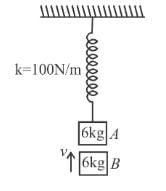Detailed Solution for Centre Of Mass NAT Level - 2 - Question 1

The initial extension in spring is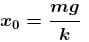Just after collision of B with A the speed of combined mass is  v/2.

For the spring to just attain natural length of combined mass must rise up by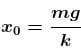(see figure.) and comes to rest.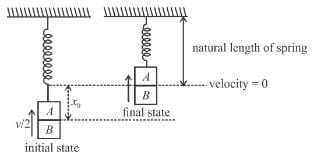Applying conservation of energy between initial and final states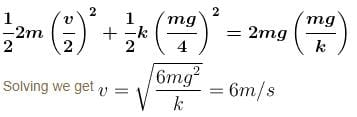Alternate solution by SHM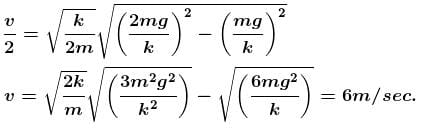*Answer can only contain numeric values
Centre Of Mass NAT Level - 2 - Question 2

### In the figure shown a small block B of mass m is released from the top of a smooth movable wedge A of the same mass m. The height of wedge A shown in figure is h = 100cm. B ascends another movable smooth wedge C of the same mass. Neglecting friction any where find the maximum height (in cm) attained by block B on wedge C.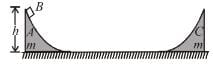Detailed Solution for Centre Of Mass NAT Level - 2 - Question 2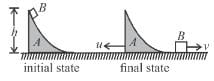Let u and v be speed of wedge A and block B at just after the block B gets off the wedge A. Applying conservation of momentum in horizontal direction, we get.

mu = mv      ...(1)

Applying conservation of energy between initial and final state as shown in figure (1), we get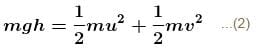solving (1) and (2) we get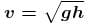...(3)

At the instant block B reaches maximum height h' on the wedge C(figure 2), the speed of block B and wedge C are v'.

Applying conservation of momentum in horizontal direction, we get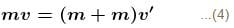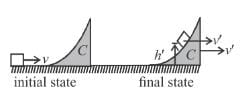Applying conservation of energy between initial and final state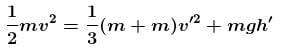Solving equations (3), (4) and (5) we get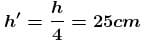*Answer can only contain numeric values
Centre Of Mass NAT Level - 2 - Question 3

### Each of the block shown in figure has mass 1 kg. The near block moves with a speed of 2 m/s towards the front block kept at rest. The spring attached to the front block is light and has a spring constant 50 N/m. Find the maximum compression of the spring. (in cm) :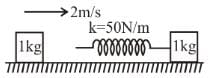Detailed Solution for Centre Of Mass NAT Level - 2 - Question 3

Maximum compression will take place when the blocks move with equal velocity. As no net external horizontal force acts on the system of the two blocks, the total linear momentum will remain constant. If v is the common speed at maximum compression, we have,

(1 kg) (2m/s) = (1kg)v + (1kg)v

or v = 1m/s

Initial kinetic energy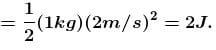Final kinetic energy =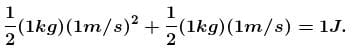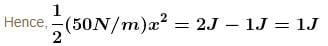or x = 0.2m

*Answer can only contain numeric values
Centre Of Mass NAT Level - 2 - Question 4

A stationary body explodes into four identical fragments such that three of them fly off mutually perpendicular to each other, each with same kinetic energy., E0. The energy of explosion is given by nE0. Find the value of n.

Detailed Solution for Centre Of Mass NAT Level - 2 - Question 4

Let the three mutually perpendicular direction be along xy and z-axis respectively.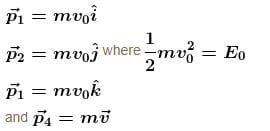By linear momentum conservation,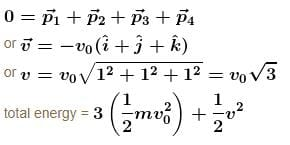= 3E0 + 3E0
= 6E0

*Answer can only contain numeric values
Centre Of Mass NAT Level - 2 - Question 5

A balloon having mass m is filled with gas and is held in hands of a boy. Then suddenly it get released and gas start coming out of it with a constant rate. The velocities of the ejected gases is also constant 2m/s with respect to the balloon. Find out the velocity of the balloon when the mass of gas is reduced to half. [Effect of atmosphere and gravity is neglected]

Detailed Solution for Centre Of Mass NAT Level - 2 - Question 5

Neglecting gravity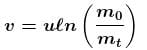u = ejection velocity w.r.t. balloon
m0 = initial mass
m1 = mass at any time t.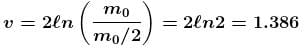*Answer can only contain numeric values
Centre Of Mass NAT Level - 2 - Question 6

Particle A moves with speed 10 m/s in a frictionless circular fixed horizontal pipe of radius 5 m and strikes with B of double mass that of A. Coefficient of restitution is 1/2 and particle A starts its journey at t = 0. The time at which second collision occurs is given by nπ sec. Find the value of n.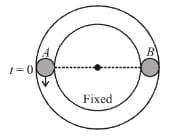Detailed Solution for Centre Of Mass NAT Level - 2 - Question 6

For first collision v = 10 m/s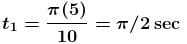velocity of separation = e velocity of approach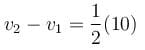v2 – v1 = 5m/s
for second collision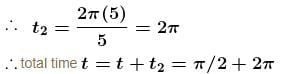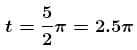*Answer can only contain numeric values
Centre Of Mass NAT Level - 2 - Question 7

A plate of mass M is moved with constant velocity v against dust particles moving with velocity u in opposite direction as shown. The density of the dust is ρ and plate area is A. Find the force F required to keep the plate moving uniformly is Aρ(u + v)N, then N is :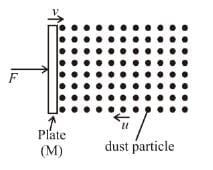Detailed Solution for Centre Of Mass NAT Level - 2 - Question 7

Force F on plate = force exerted by dust particles
= force on dust particles by the plate
= rate of change of momentum of dust particles
= mass of dust particles striking the plate per unit time × change in velocity of dust particles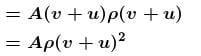*Answer can only contain numeric values
Centre Of Mass NAT Level - 2 - Question 8

Three ball AB and C (mA = mC = 4 mB) are placed on a smooth horizontal surface. Ball B collides with ball C with an initial velocity v as shown in the figure. Total number of collision between the balls will be : (All collisions are elastic)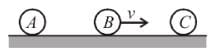Detailed Solution for Centre Of Mass NAT Level - 2 - Question 8

For 1st collision
Since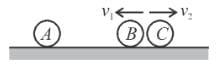e = 1
v = v1 + v2
⇒ v2 = v – v1   ...(1)
By momentum conservation :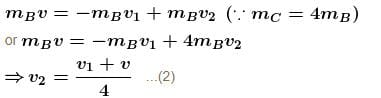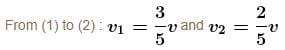For second collision :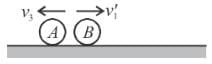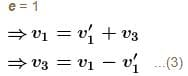By momentum conservation :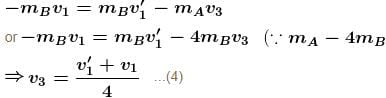From (2) and (4) :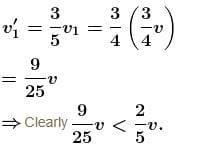Therefore B can not collide with C for the second time.
Hence; total number of collision is 2.

*Answer can only contain numeric values
Centre Of Mass NAT Level - 2 - Question 9

A particle moving on a smooth horizontal surface strikes a stationary wall. The angle of strikes is equal to the angle of rebound and is equal to 37° and the coefficient of restitution with wall is e = 1/5. Find the friction coefficient between wall and the particle in the form X/10. What is the value of X.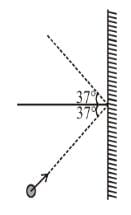Detailed Solution for Centre Of Mass NAT Level - 2 - Question 9

Since e = 1/5

∴  Final normal component of velocity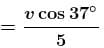As the angle of rebound is equal to the angle before impact.

Therefore, both normal and tangential components of velocities must change by the same factor,
∴ Tangential velocity after impact becomes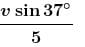Let the time of impact be Δt.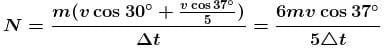where N is the normal force imparted on the ball by the wall.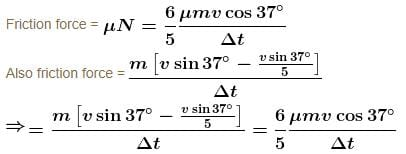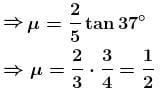*Answer can only contain numeric values
Centre Of Mass NAT Level - 2 - Question 10

Three blocks are placed on smooth horizontal surface and lie on same horizontal straight line. Block 1 and block 3 have mass m each and block 2 has mass M(M > m). Block 2 and block 3 are initially stationary, while block 1 is initially moving towards block 2 with speed v as shown. Assume that all collisions are headon and perfectly elastic. What value of M/m ensures that block 1 and block 3 have the same final speed?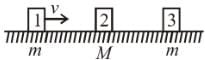Detailed Solution for Centre Of Mass NAT Level - 2 - Question 10

Let v1v2 and v3  be velocities of block 1, 2 and 3 after suffering collision each.
mv = mv1 + Mv2
and v1 – v2 = –v
v1 – v2 = –v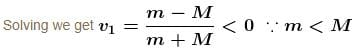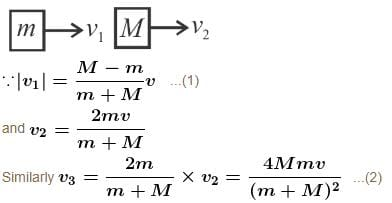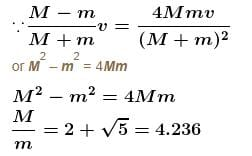## Topic wise Tests for IIT JAM Physics

217 tests
 Use Code STAYHOME200 and get INR 200 additional OFF Use Coupon Code
Information about Centre Of Mass NAT Level - 2 Page
In this test you can find the Exam questions for Centre Of Mass NAT Level - 2 solved & explained in the simplest way possible. Besides giving Questions and answers for Centre Of Mass NAT Level - 2, EduRev gives you an ample number of Online tests for practice

217 tests